Author: Oscar Cronquist Article last updated on April 06, 2022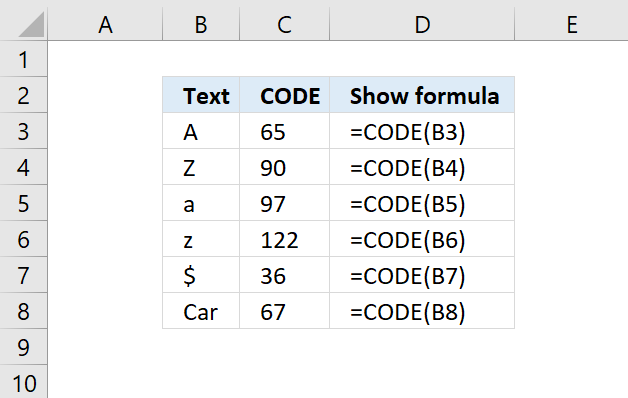The CODE function returns a specific number for the first character of the text argument, determined by your computer's character set.

 Windows ANSI Macintosh Macintosh character set

CODE(text)

## 2. CODE Function Arguments

 text Required. The CODEacter for which you want the corresponding code.

## 3. CODE Function example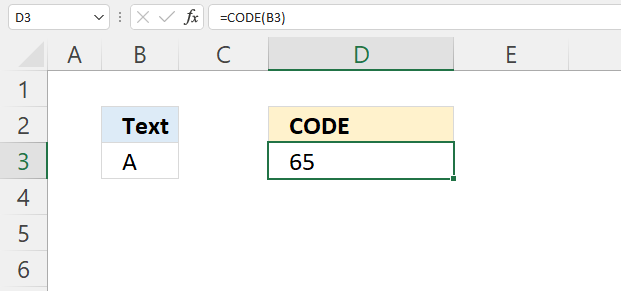Formula in cell D3:

=CODE(B3)

### 3.1 Explaining formula

CODE(B3)

becomes

CODE("A")

and returns 65.

A to Z corresponds to numbers 65 to 90. a to z corresponds to numbers 97 to 122.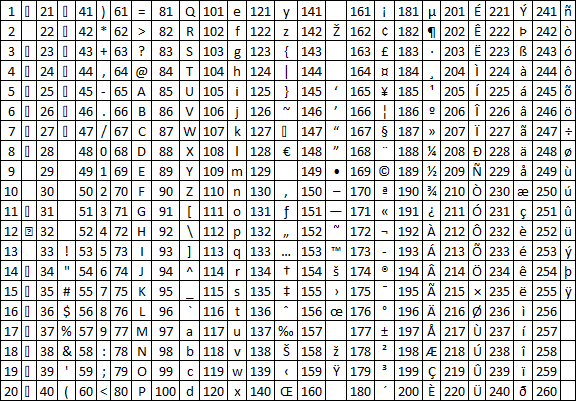## 4. CODE Function - convert value to ANSI numbers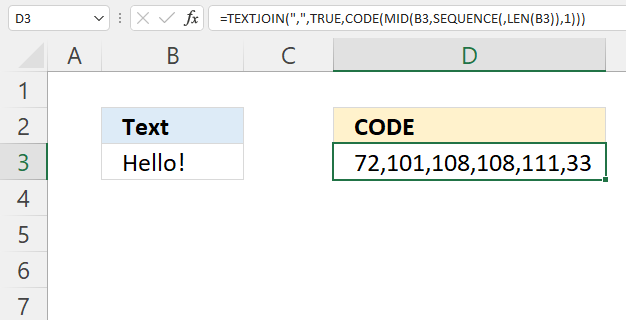Formula in cell D3:

=TEXTJOIN(",",TRUE,CODE(MID(B3,SEQUENCE(,LEN(B3)),1)))

### Explaining formula

#### Step 1 - Count characters in cell

The LEN function returns the number of characters in a cell value.

LEN(value)

LEN(B3)

becomes

LEN("Hello!")

and returns 6. There are six characters in cell B3.

#### Step 2 - Create numbers from 1 to n

The SEQUENCE function creates a list of sequential numbers.

SEQUENCE(rows, [columns], [start], [step])

SEQUENCE(,LEN(B3))

becomes

SEQUENCE(,6)

and returns {1, 2, 3, 4, 5, 6}.

#### Step 3 - Split characters in cell

The MID function returns a substring from a string based on the starting position and the number of characters you want to extract.

MID(textstart_numnum_chars)

MID(B3,SEQUENCE(,LEN(B3)),1)

becomes

MID("Hello!",{1, 2, 3, 4, 5, 6},1)

and returns {"H","e","l","l","o","!"}.

#### Step 4 - Convert characters to numbers

CODE(MID(B3,SEQUENCE(,LEN(B3)),1))

becomes

CODE({"H","e","l","l","o","!"})

and returns {72,101,108,108,111,33}.

#### Step 5 - Join strings

The TEXTJOIN function allows you to combine text strings from multiple cell ranges and also use delimiting characters.

TEXTJOIN(delimiterignore_emptytext1[text2], ...)

TEXTJOIN(",",TRUE,CODE(MID(B3,SEQUENCE(,LEN(B3)),1)))

becomes

TEXTJOIN(",",TRUE,{72,101,108,108,111,33})

and returns

"72,101,108,108,111,33".

## 5. CODE Function all characters

 2  34 " 66 B 98 130 ‚ 162 194 Â 226 â 3  35 # 67 C 99 131 ƒ 163 195 Ã 227 ã 4  36 \$ 68 D 100 132 „ 164 196 Ä 228 ä 5  37 % 69 E 101 133 … 165 197 Å 229 å 6  38 & 70 F 102 134 † 166 198 Æ 230 æ 7  39 ' 71 G 103 135 ‡ 167 199 Ç 231 ç 8  40 ( 72 H 104 136 ˆ 168 200 È 232 è 9 41 ) 73 I 105 137 ‰ 169 201 É 233 é 10 42 * 74 J 106 138 Š 170 202 Ê 234 ê 11 43 + 75 K 107 139 ‹ 171 203 Ë 235 ë 12 44 , 76 L 108 140 Œ 172 204 Ì 236 ì 13 45 - 77 M 109 141  173 205 Í 237 í 14  46 . 78 N 110 142 Ž 174 206 Î 238 î 15  47 / 79 O 111 143  175 207 Ï 239 ï 16  48 0 80 P 112 144  176 208 Ð 240 ð 17  49 1 81 Q 113 145 ‘ 177 209 Ñ 241 ñ 18  50 2 82 R 114 146 ’ 178 210 Ò 242 ò 19  51 3 83 S 115 147 “ 179 211 Ó 243 ó 20  52 4 84 T 116 148 ” 180 212 Ô 244 ô 21  53 5 85 U 117 149 • 181 213 Õ 245 õ 22  54 6 86 V 118 150 – 182 214 Ö 246 ö 23  55 7 87 W 119 151 — 183 215 × 247 ÷ 24  56 8 88 X 120 152 ˜ 184 216 Ø 248 ø 25  57 9 89 Y 121 153 ™ 185 217 Ù 249 ù 26  58 : 90 Z 122 154 š 186 218 Ú 250 ú 27  59 ; 91 [ 123 155 › 187 219 Û 251 û 28 60 < 92 \ 124 156 œ 188 220 Ü 252 ü 29 61 = 93 ] 125 157  189 221 Ý 253 ý 30 62 > 94 ^ 126 158 ž 190 222 Þ 254 þ 31 63 ? 95 _ 127 159 Ÿ 191 223 ß 255 ÿ 32 64 @ 96 ` 128 160 192 224 à 256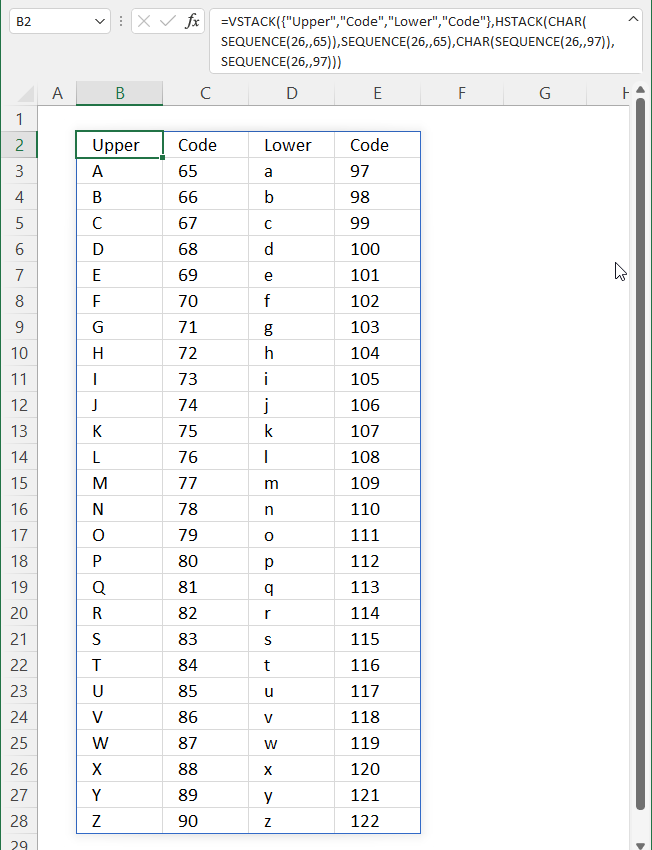The image above shows upper and lower letters and their corresponding number, here is the formula:

=VSTACK({"Upper", "Code", "Lower", "Code"}, HSTACK(CHAR(SEQUENCE(26, , 65)), SEQUENCE(26, , 65), CHAR(SEQUENCE(26, , 97)), SEQUENCE(26, , 97)))

### Explaining formula

#### Step 1 - Create numbers from 65 to 90

The SEQUENCE function creates a list of sequential numbers.

SEQUENCE(rows, [columns], [start], [step])

SEQUENCE(26, , 65)

returns {65;66;67; ... ; 90}

#### Step 2 - Create characters

The CHAR function converts a number to the corresponding ANSI character determined by your computers character set.

CHAR(number)

CHAR(SEQUENCE(26, , 65))

becomes

CHAR({65;66;67; ... ; 90})

and returns {"A";"B";"C"; ... ; "Z"}

#### Step 3 - Join arrays horizontally

The HSTACK function lets you combine cell ranges or arrays, it joins data to the first blank cell to the right of a cell range or array (horizontal stacking)

HSTACK(array1,[array2],...)

HSTACK(CHAR(SEQUENCE(26, , 65)), SEQUENCE(26, , 65), CHAR(SEQUENCE(26, , 97)), SEQUENCE(26, , 97))

becomes

HSTACK({"A";"B";"C"; ... ; "Z"}, {65;66;67; ... ; 90}, {"a";"b";"c"; ... ; "z"}, {97;98;99; ... ; 122})

and returns

{"A",65,"a",97;"B", ... , "z",122}

The VSTACK function lets you combine cell ranges or arrays, it joins data to the first blank cell at the bottom of a cell range or array.

VSTACK(array1,[array2],...)

VSTACK({"Upper", "Code", "Lower", "Code"}, HSTACK(CHAR(SEQUENCE(26, , 65)), SEQUENCE(26, , 65), CHAR(SEQUENCE(26, , 97)), SEQUENCE(26, , 97)))

becomes

VSTACK({"Upper", "Code", "Lower", "Code"}, {"A",65,"a",97;"B", ... , "z",122})

and returns

"Upper","Code","Lower","Code";"A",65, ... ,"z",122}.

## 6. CODE function - returns #VALUE!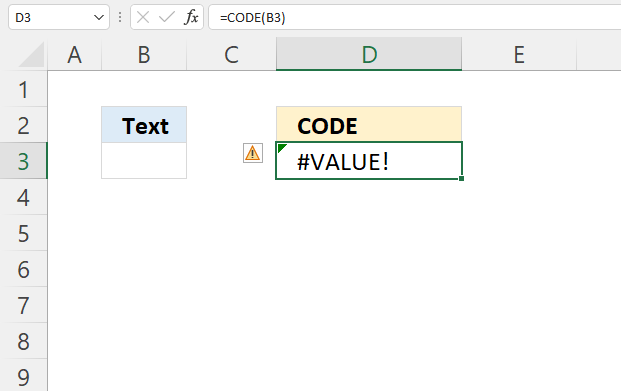The CODE function returns a #VALUE! error if the cell is empty.

Formula in cell D3:

=CODE(B3)

### Explaining formula

CODE(B3)

becomes

CODE("")

and returns #VALUE! error.